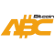Bitcoin ABC  0.22.12 P2P Digital Currency
amount.h File Reference
`#include <serialize.h>`
`#include <cstdlib>`
`#include <ostream>`
`#include <string>`
`#include <type_traits>`
Include dependency graph for amount.h:
This graph shows which files directly or indirectly include this file:

Go to the source code of this file.

struct  Amount

## Functions

bool MoneyRange (const Amount nValue)

## Variables

static constexpr Amount SATOSHI = Amount::satoshi()

static constexpr Amount CASH = 100 * SATOSHI

static constexpr Amount COIN = 100000000 * SATOSHI

static const Amount MAX_MONEY = 21000000 * COIN
No amount larger than this (in satoshi) is valid. More...

## ◆ MoneyRange()

 bool MoneyRange ( const Amount nValue )
inline

Definition at line 166 of file amount.h.

Here is the call graph for this function:
Here is the caller graph for this function:

## ◆ CASH

 constexpr Amount CASH = 100 * SATOSHI
static

Definition at line 152 of file amount.h.

## ◆ COIN

 constexpr Amount COIN = 100000000 * SATOSHI
static

Definition at line 153 of file amount.h.

## ◆ MAX_MONEY

 const Amount MAX_MONEY = 21000000 * COIN
static

No amount larger than this (in satoshi) is valid.

Note that this constant is not the total money supply, which in Bitcoin currently happens to be less than 21,000,000 BCH for various reasons, but rather a sanity check. As this sanity check is used by consensus-critical validation code, the exact value of the MAX_MONEY constant is consensus critical; in unusual circumstances like a(nother) overflow bug that allowed for the creation of coins out of thin air modification could lead to a fork.

Definition at line 165 of file amount.h.

## ◆ SATOSHI

 constexpr Amount SATOSHI = Amount::satoshi()
static

Definition at line 151 of file amount.h.